Analysis WebNotes
arrow_back arrow_forward

# Class Contents

## Convergence and Continuity in Euclidean Spaces

We start with a very straightforward function. We would hope there's nothing difficult about showing that a function like this is continuous.

Example: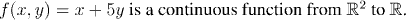Question: Write down exactly what the statement "f(x,y) is continuous" means, when all the Euclidean norms have been expanded.

This highlights how hard it can be to use the Euclidean metric to prove continuity directly. Another example underscores the point even more dramatically.

Example: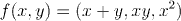Question: Again, write down exactly what the statement "f(x,y) is continuous" means, when all the Euclidean norms have been expanded.

Now we develop a more systematic way of proving continuity. The key is to be able to separate the individual real variables x and y out of the variable x=(x,y) in R2.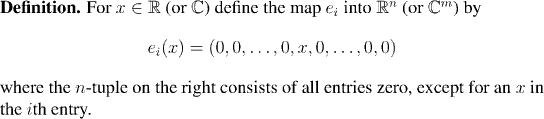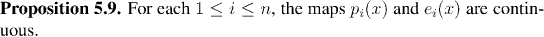Now let's see how to use these two functions to prove that one of our previous examples is continuous.

Example:

The functionis continuous.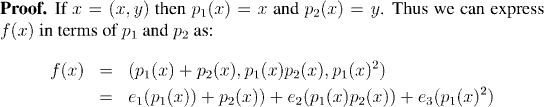The kind of results on arithmetic of continuous functions that we've been collecting all along ought to come in here to prove that sums and products of continuous function are continuous. Let's see exactly what we need.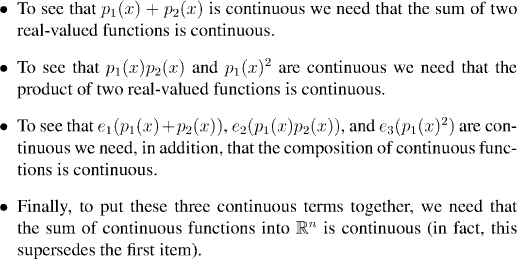The next two propositions are concerned with proving the necessary technical details to complete these outstanding points.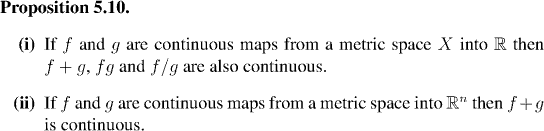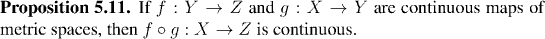We can finish off the discussion of the last example now: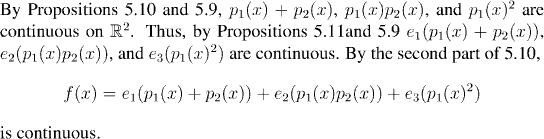Now let's have a couple more examples of how to use the maps pi and ei to show that functions are continuous.

Example:Example: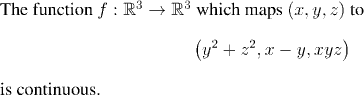Back in Chapters 2 and 3 we introduced the idea of open and closed sets of real numbers. In the next section we study open and closed sets in general metric spaces. This will prove to be a very fruitful concept, and we will find that we already know how to prove most of the theorems, from our experience of the real case.